• 不动点迭代法（Fixed point iteration method） 原理网上已经很多了，这里不再赘述。 需要注意的是不动点迭代法的使用条件。 举个简单的例子，利用不动点法求解方程f(x) = 1 + 0.5*sin(x) − x = 0在（1,2）区间的根...
不动点迭代法（Fixed point iteration method）
原理网上已经很多了，这里不再赘述。 需要注意的是不动点迭代法的使用条件。
举个简单的例子，利用不动点法求解方程f(x) = 1 + 0.5*sin(x) − x = 0在（1,2）区间的根。 令g(x)=1+0.5*sin(x)

g = @(x) fun2(x);
% Initialization
x0 = 0;
tol = 1e-5;
maxIter = 40;

% Test biSection function
[xStar,xRoot] = fixPoint(g,x0,tol,maxIter);
fprintf('The fixed point is: %d\n',xStar);
fprintf('The root of the equation is: %d\n',xRoot);

function [xStar,xRoot] = fixPoint(fun2,x0,tol,maxIter)
% Inputs:
% fun2: a function handle, standing for the function written above
% x0: the initial guess of the fixed point
% tol: the tolerance within which the program can stop
% maxIter: the maximum number of iterations the program is allowed to run
% Outputs:
% xStar: the numerical value of the fixed point
% xRoot: the numerical value of the root

x = zeros(maxIter,1);
x(1) = fun2(x0);
i = 1;

while abs(fun2(x(i))-x(i))>tol && i<maxIter
x(i+1) = fun2(x(i));
xStar = x(i);
i = i+1;
end
xRoot = x(i);

end

function gx = fun2(x)
gx = 1+0.5*sin(x);
end


展开全文matlab
• 不动点迭代法解非线性方程的aitken加速法matlab实现
• 1. 不动点和不动点迭代法设 f 是连续函数，为了解方程 把方程变换为等价的方程 其中φ 是连续函数。若x* 是f 的零点，即f(x*)=0 则有 x*称为函数φ 的一个不动点.构造的迭代法 这称为一种...
1. 不动点和不动点迭代法
设 f 是连续函数，为了解方程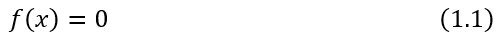把方程变换为等价的方程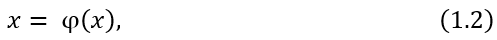其中φ 是连续函数。若x* 是f 的零点，即f(x*)=0 则有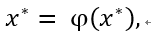x*称为函数φ 的一个不动点.构造的迭代法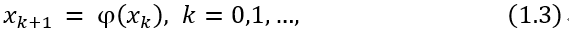这称为一种不动点迭代法， 称为迭代函数。由(1.3)式产生的序列 {xk}如果满足 ,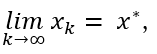则 x*是φ的一个不动点。即方程(1.1)的一个根。
2.函数在区间上不动点的存在性和唯一性及推论
定理 2.1    设 φ∈C[a,b]且满足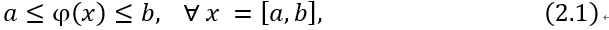则 φ 在[a,b] 上一定存在不动点。若φ 满足(2.1),又存在常数L∈(0,1) 使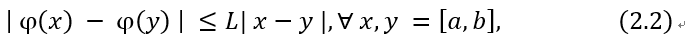则φ 在[a,b] 的不动点是唯一的。
条件 (2.2)称为Lipschitz条件，L称为Lipschitz常数。

定理 2.1的推论 设函数φ满足(2.1)，又 φ'∈C[a,b]，且存在常数L∈(0,1) ，使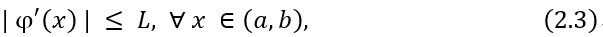则φ 在[a,b] 上存在唯一的不动点。
3.迭代法在区间[a,b]的收敛性
定理3.1    设φ∈C[a,b]，满足条件(2.1)和(2.2),其中L∈(0,1)。则对任意的x0∈[a,b]，由迭代法(1.3) 产生的序列{xk}收敛到φ在[a,b]上的不动点x* ,而且对整数P≥1，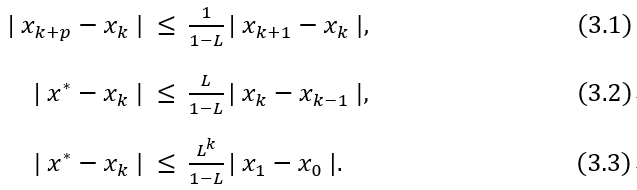定理3.1描述了对任意的x0∈[a,b]，{xk}收敛到[a,b]唯一的不动点x*，这可以说是在区间[a,b]上的全局收敛性。
局部收敛性
定义 设函数φ在区间[a,b]上有不动点x* , 如果存在x*的一个邻域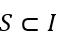对任意的 x0∈S，迭代法(1.3)产生的序列{xk}∈S，且{xk}收敛到x*，就称迭代法(1.3)局部收敛。

定理3.2   设 x*为函数φ 的不动点， 在φ'在x*的某个邻域上存在且连续，且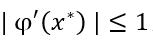则迭代法(1.3)局部收敛。
4.牛顿迭代法
设x*是f的零点，并已有一个近似值xk≈x*，如果f''存在且连续，由Taylor展开式得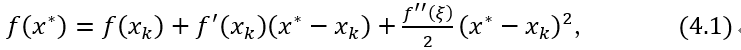,
其中ξ在xk和x*之间。因为f(x*)=0，如果f'(xk)≠ 0，略去最后一项即得
.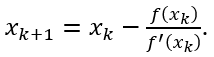这就是Newton迭代法，又称Newton-Raphson迭代法。
牛顿迭代法的局部收敛性
Newton迭代法对应的迭代函数是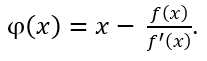定理4.1    设f(x*)=0 ，f'(x*)≠ 0，且f在包含x*的一个区间上有二阶连续导数，则牛顿迭代法(4.2)局部收敛到x* 。

练习题：设 a＞0, 求平方根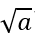可以转化为解方程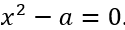求迭代公式及迭代公式在(0,+∞)上的全局收敛性。并和二分法比较。
练习题解答请参考：点击打开链接

5.参考文献最优化理论与算法(第二版).陈宝林编著.


展开全文最优化方法
• 我的机器学习教程「美团」算法工程师带你入门机器学习 已经开始更新了，欢迎大家订阅~ 任何关于算法、编程、AI行业知识或博客内容的问题，可以随时扫码关注公众号「图灵的猫」，加入”学习小组“，沙雕博主在线答疑...
任何关于算法、编程、AI行业知识或博客内容的问题，可以随时扫码关注公众号「图灵的猫」，加入”学习小组“，沙雕博主在线答疑~此外，公众号内还有更多AI、算法、编程和大数据知识分享，以及免费的SSR节点和学习资料。其他平台（知乎/B站）也是同名「图灵的猫」，不要迷路哦~1、不动点（FixedPoint）
首先来看一下什么是不动点【1】：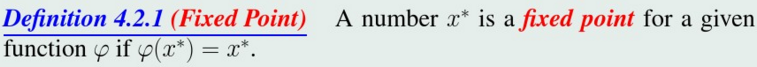换句话说，函数φ的不动点是y=φ(x)与y=x的交点，下图画出了函数y=cos(x)与y=x在区间[0,π/2]的交点，即cos(x)的不动点【2】：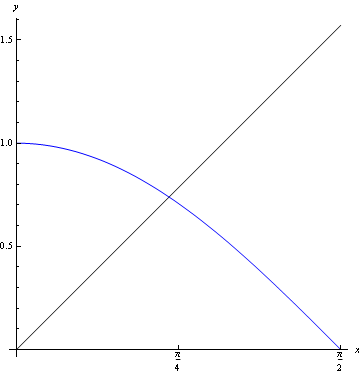2、不动点迭代（Fixed Point Iteration）
不动点迭代又称为简单迭代（simple iteration）。下面来看一下不动点迭代【3】：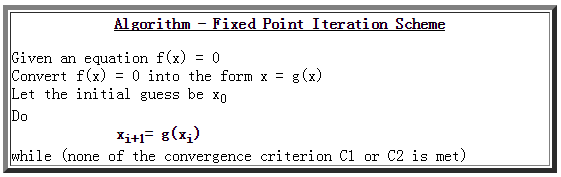也就是说，为了求解方程f(x)=0，首先将方程转换为x=g(x)，然后初始化x0，循环迭代xi+1=g(xi)，直到满足收敛收件。
这里将方程f(x)=0转换为x=g(x)是很容易的，比如对于f(x)=x-cos(x)，求解f(x)=0即为求解x-cos(x)=0，即x=cos(x)，因此g(x)=cos(x)；再例如对于方程【4】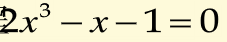可以等价为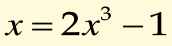还可以等价为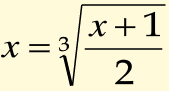也就是说，将方程f(x)=0转换为x=g(x)有不同的方式，因此对方程f(x)=0来说，g(x)也不是唯一的。
3、不动点迭代的收敛性
这个迭代过程是很简单的，但这里有个关键性的问题：迭代收敛么？即经过N次迭代后是否会收敛于不动点？
3.1 例子
先看两个例子，这里有两个方程【5】：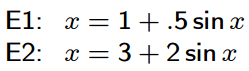可以通过其它方法得到方程E1和E2的根：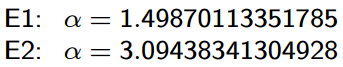画出E1和E2曲线：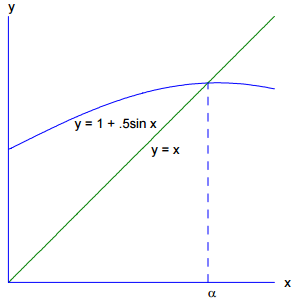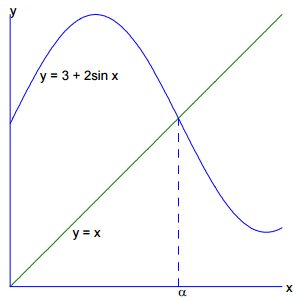3.2 不动点迭代MATLAB程序
为了运用不动点迭代求E1和E2的根，我编写了如下MATLAB程序：

[plain]
view plain
copy

function [y] = FixedPointIter(x0,func,tol,MaxIter)  % Version: 1.0 written by jbb0523 @2016-08-21      if nargin < 4          MaxIter = 100;      end      if nargin < 3          tol = 1e-3;      end      xn = x0;      fprintf('Iter  0: %16.14f\n',x0);      xnp1 = func(xn);      fprintf('Iter  1: %16.14f\n',xnp1);      criterion = abs(xnp1-xn);      xn = xnp1;      Iter = 1;      while(criterion>tol)          xnp1 = func(xn);          criterion = abs(xnp1-xn);          xn = xnp1;          Iter = Iter + 1;          fprintf('Iter %2.0d: %16.14f\n',Iter,xnp1);          if Iter>=MaxIter              break;          end      end      y = xnp1;  end

3.3 例子测试与分析
分别在CommandWindow中执行以下两条命令：

[plain]
view plain
copy

FixedPointIter(0,@E1,1e-12,10);

[plain]
view plain
copy

FixedPointIter(3,@E2,1e-12,10);

注意，以上两条命令分别调用了E1和E2函数：

[plain]
view plain
copy

function [y] = E1(x)      y = 1+0.5*sin(x);  end

[plain]
view plain
copy

function [y] = E2(x)      y = 3+2*sin(x);  end

可对E1和E2分别执行10次不动点迭代：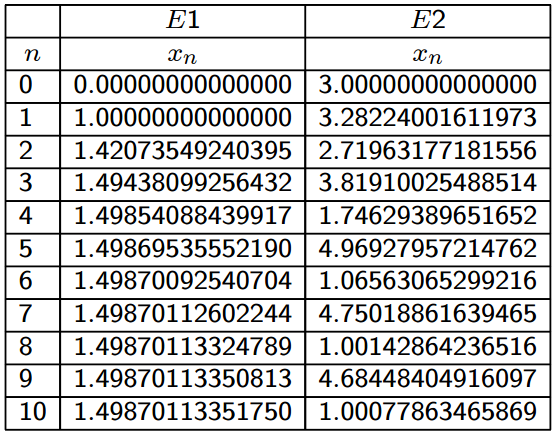从迭代过程来看，对E1执行不动点迭代后收敛了，而对E2执行不动点迭代后明显发散了。
那么什么时候不动点迭代收敛，而又什么时候不动点迭代发散呢？
注意起始点的设定，对于E1来讲，其根约为1.4987，迭代时初始化为0（这个初始点与根比较远），而最终收敛到了约1.4987，而对于E2来讲，其根约为3.0945，迭代时初始化为3（这个初始点与根很接近），但最终发散了。
因此，这不能怪起始点不照顾E2……
观察E1和E2曲线，我们大概可以得到一个直觉，E1在不动点处曲线较为平坦，而E2在不动点处曲线较为陡峭。
3.4 不动点迭代收敛定理
下面给出有关不动点迭代收敛性的定理【4】：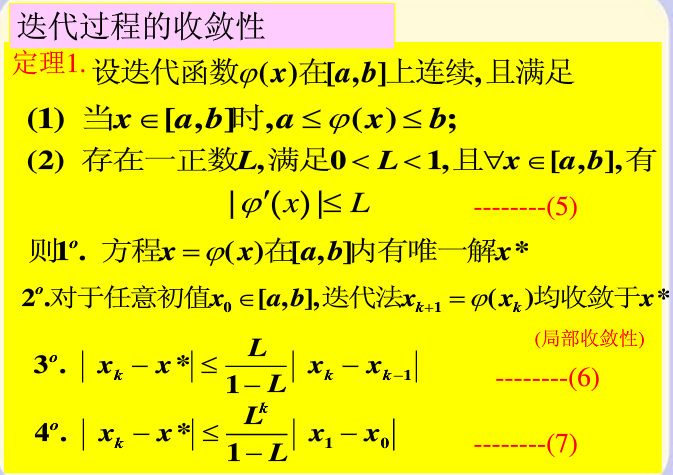通俗点讲，若要使不动点迭代收敛，则要求φ(x)在区间[a,b]上的函数值也在此区间内，另外还要求φ(x)的斜率绝对值不大于1。其证明过程比较复杂，有兴趣的可以查阅一些相关文献。
应用此定理，可以来解释两个例子中为什么E1收敛而E2发散【5】：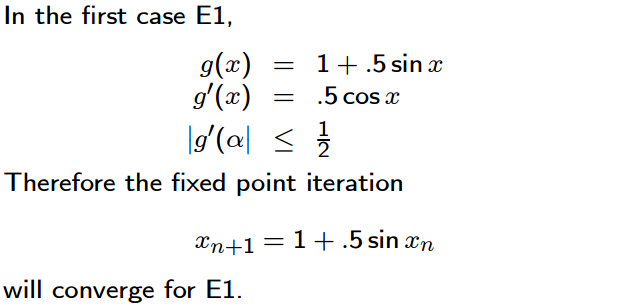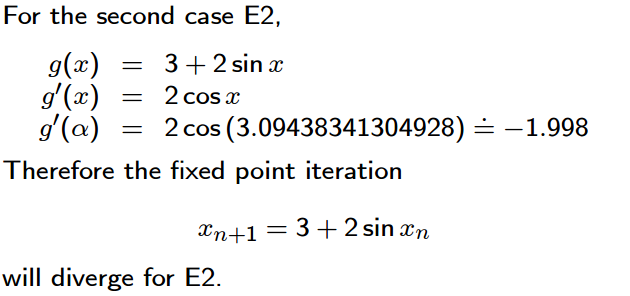我们可以通过下图来直观的感觉一下不动点迭代收敛的过程【2】：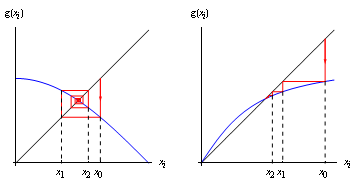对于左图，斜率小于零，迭代路径是一圈一圈的缩小；对于右图，斜率大于零，迭代路径是直接折线式逼近不动点。
我们再通过下图来直观的感觉一下不动点迭代发散的过程【2】：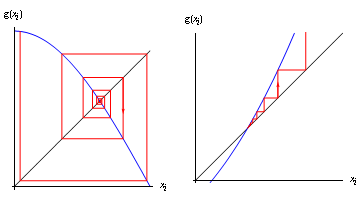对于左图，斜率小于零，迭代路径是一圈一圈的变大；对于右图，斜率大于零，迭代路径是直接折线式远离不动点。
注意看上图时与迭代过程相结合才能看明白，先给定x0，得到x1=φ(x0)，再得到x2=φ(x1)，依此类推……
4、结束语
其实本来是在看SpaRSA（后面再说），里面有个Continuation，然后就查到了FPC，一直很好奇FPC为什么要Fixed Point Continuation，虽然FPC文献中一直提到Fixed Point Iteration和Fixed Point Equation，但也也没注意，后来看到了【6】，里面再一次提到了Fixed Point Iteration，难道Fixed Point Iteration是一个数学问题么？于是才开始百度……
压缩感知凸优化类重构算法这个坑太大，每一个算法的背后都有一堆剪不断理还乱的数学问题……
继续前行吧，还是那句话：路会越走越宽的……
5、参考文献
【1】百度文库：4.2Fixed-Point Iteration
【2】http://www.imperial.ac.uk/：NumericalMethods: Fixed Point Iteration
【3】mat.iitm.ac.in/：FIXEDPOINT ITERATION METHOD
【4】百度文库：6.2不动点迭代法及其收敛定理
【5】https://uiowa.edu/：FIXEDPOINT ITERATION
【6】http://www.maths.lth.se/na/courses/FMN081/FMN081-06/lecture6.pdf
展开全文泛函分析 拓扑 机器学习 统计学习
• 在[a,b]区间内寻找方程x**5-2*x-1=0的根的初始近似值位置，确定不动点迭代的初始点（可能有多个），然后使用不动点迭代法求方程的根（可能有多个根）。前后两次迭代的差的绝对值小于delta后停止迭代。 输入形式 在...
问题描述
在[a,b]区间内寻找方程x**5-2*x-1=0的根的初始近似值位置，确定不动点迭代的初始点（可能有多个），然后使用不动点迭代法求方程的根（可能有多个根）。前后两次迭代的差的绝对值小于delta后停止迭代。
输入形式
在屏幕上输入3个数，依次为区间左端点值a、右端点值b和所求根的精度值。各数间都以一个空格分隔。根据输入的所求根的精度值可求得delta.
输出形式
每一行输出一个根（精确到小数点后3位）
样例1输入
-1.2 1.5 3
样例1输出
-1.000 -0.519 1.291
样例1说明
输入：左端点a值为-1.2，右端点b值为1.5，前后两次迭代的差的绝对值小于delta=10**(-3)后停止迭代。输出：从小到大顺序输出三个根的值。
代码
import numpy as np

def f(x):
return x ** 5 - 2 * x - 1

def g1(x):
return (x**5-1)/2

def g2(x):
result = (abs(2 * x + 1))**(1 / 5)
if (2 * x - 1) < 0:
return -result
return result

def getEpsilon(x, epsilon):
maxY = minY = x
for each in x:
maxY = max(f(each), maxY)
minY = min((f(each), minY))
epsilon = (maxY - minY) * epsilon
return epsilon

def getInitialVal(x, N, step, epsilon):
initalVal = []
for i in range(N + 1):
y1, y2, y3 = f(x - step), f(x), f(x + step)
if (y1 * y2 < 0) and (i != 0):
initalVal.append((x + x - step) / 2)
if ((y2 - y1) * (y3 - y2) < 0) and (abs(y2) < epsilon):
initalVal.append(x)
x += step

return initalVal

def findFixedPoint(initalVal, delta,epsilon):
points = []
for each in initalVal:
if (abs(g1(each)) < 1):
points.append(iteration(each, g1, delta,epsilon))
else:
points.append(iteration(each, g2, delta,epsilon))
return points

def iteration(p1, g, delta,epsilon):
while True:
p2 = g(p1)
err =abs(p2-p1)
relerr = err/(abs(p2)+epsilon)
if err<delta or relerr<delta:
return p2
p1 = p2

def main():
a, b, c = input().split(' ')
a = float(a)
b = float(b)
c = int(c)
delta = 10 ** (-c)
N = 8
epsilon = 0.01
step = (b - a) / N
x = np.arange(a, b + epsilon, epsilon)

epsilon2 = getEpsilon(x,epsilon)
initalVal = getInitialVal(a, N, step, epsilon2)
ans = findFixedPoint(initalVal, delta,epsilon)

for each in ans:
print('%.3f' % each)

if __name__ == '__main__':
main()

展开全文python
• 不动点迭代法的实现 不动点迭代法，是求方程的迭代方法。为什么要迭代的求，直接法不好吗？直接法显然比较好，但是存在弊端，比如函数形式较复杂时，求解器不容易直接求得。利用不动点的性质，可以...
• 分裂共同半压缩映射不动点问题是一类比较经典的问题模型,目前算法多是运用当前迭代点的信息构建新的迭代点,这类算法收敛比较慢,且仅具有线性收敛性。为构建快速有效算法,受惯性近似算法求解极大单调算子零点问题的...
• 针对航空航天领域中存在的控制分配问题, 提出一种基于最大方向导数(MDD) 不动点迭代的控制分配策 略. 定义MDD为当前迭代点沿所有单位向量方向中最大导数对应的单位向量, 沿MDD方向, 取前一迭代点和当前 ...
• 在对第4题第3问进行分析的过程中，发现matlab程序能够更加直观的求解计算这个问题。 clc; clear; x=2; m=0.00001; N=60; for k=1:N y=x-1/2/sqrt(3)*(x^2-3); if (abs(x-y))>...程序中x（迭代初始值）,mmatlab
• 方程求根：二分法--不动点迭代--牛顿--弦截1.问题概述 许多复杂的求解问题，都可以转换成方程f(x)=0的求解问题。这一系列的解叫做方程的根。对于非线性方程的求解，在自变量范围内往往有多个解，我们将此变化...方程求根 二分法
• 这一节则主要是针对算法的收敛性进行分析，试图从一个更加抽象的层面，利用不动点迭代的思想，把上面的算法综合起来，给一个比较 general 的收敛性分析方法。 1. 什么是不动点？ 对于希尔伯特空间(Hilbert space) H\...收敛性
• ## 不动点迭代（Fixed Point Iteration）

万次阅读 多人点赞 2016-09-07 15:30:14
之所以学习不动点迭代是由于近来看到了FPC算法，即Fixed PointContinuation，有关FPC后面再详述。  从搜索到的资料来看，不动点迭代是一个很基本很常见的概念，具体出自哪一门基础课不详，反正之前我没听说过。
• 关于不动点迭代和二分法、试值的本质区别，在[上一篇介绍二分法、试值]的博客中提到了(https://blog.csdn.net/weixin_42572826/article/details/104934857)，理论上不动点迭代，只能对给定的一个近似解迭代找到...二分法 python
• 近年来,学者们对映像族的不动点的研究越来越活跃,迭代格式也越来越丰富,但大多都是对非扩张映像族的不动点迭代法进行的研究,而且有很多算法比较繁琐。为了寻求一种更好的算法来逼近拟非扩张影像族的不动点,在实...
• 上一课我们介绍了算法模式中的分治法，这一课继续介绍迭代法，我们一般在求解一个问题的时候，都是使用明确的方法或计算公式，带入已知量，一次性求得问题的解。但是如果用计算机解决这些问题，常常因为各种原因无法...
• 本节书摘来自华章出版社《数值...1.2　不动点迭代 使用计算器或者计算机以一个任意初值开始不断计算函数cos.也就是说，对于一个任意初值应用cos函数，然后对于结果再计算cos，然后得到一个新结果，周而复始.（如果...
• 本科课程参见：《软件学院那些课》 牛顿迭代公式 设已知方程f(x)=0的近似根x0 ,则在x0附近f(x)可用一阶泰勒多项式近似代替....用x1表示p(x)=0的根,它...这就是著名的牛顿迭代公式,它相应的不动点方程为 Jaco...
• ## 迭代优化算法

万次阅读 2016-10-14 13:47:24
如果学习机器学习算法，你会发现，其实机器学习的过程大概就是定义一个模型的目标函数J(θ)，然后通过优化算法从数据中求取J(θ)取得极值时对应模型参数θ的过程，而学习到的参数就对应于机器学习到的知识。...
• 牛顿迭代公式 设已知方程f(x)=0的近似根x0 ,则在x0附近f(x)可用一阶泰勒多项式近似代替.... 方程f(x)=0可近似地表示为p(x)=0。用x1表示p(x)=0的根,它与f(x)=0的根...这就是著名的牛顿迭代公式,它相应的不动点方程为解方程 Newton
• 牛顿迭代法本质上是一种特殊的不动点迭代，只不过它的迭代函数的构造比较特殊，所以就代码上来看，和不动点迭代法求根的是完全一样的，所不同的是，其输入的不动点迭代函数满足f_x=x-f(x)/f’(x).下面是简要证明 ...matlab 程序人生
• http://blog.csdn.net/pipisorry/article/details/24574293牛顿和拟牛顿（Newton's method & Quasi-Newton Methods）牛顿（Newton's method）　又称为牛顿-拉弗森方法（Newton-Raphson method），单变量下又...最优化方法
•平方根 素数 切线法
• 动态聚类算法：先选取初始的中心（每个类别的初始中心），然后把所有的样本进行聚类分析，聚类完成后，就去判断这个聚类结果合合理（满满足设计指标要求），如果合理就输出聚类结果（样本分类结果），如果合理...模式识别
• ## 如何通俗易懂地讲解牛顿迭代法？

万次阅读 多人点赞 2018-08-19 13:02:37
五次及以上多项式方程没有根式解（就是没有像二次方程那样的万能公式），这个...没有根式解意味着方程解出来，数学家也提供了很多方法，牛顿迭代法就是其中一种。 1 切线是曲线的线性逼近 要讲牛顿迭代法之前...
• ## 迭代法 递归 区别

千次阅读 2012-05-10 20:31:50
迭代法 求助编辑   迭代法 迭代法也称辗转法，是一种不断用变量的旧值递推新值的过程,跟迭代法相对应的是直接法（或者称为一次解法），即一次性解决问题。迭代法又分为精确迭代和近似...recursion input 编程 数据结构
• 基于有自由面渗流分析的高斯点,建立了求解渗流问题的非光滑非线性方程组模型和求解此类问题的有限元混合不动点算法,此方法属于固定网格法,只需划分一次网格,...对不动点法的收敛性分析为迭代法的收敛提供了理论依据 。
• 研究生课程系列文章参见索引《在...解决这个问题使用的最多的方法是迭代最近点法（Iterative Closest Points Algorithm）。 基本思想是：根据某种几何特性对数据进行匹配，并设这些匹配为假想的对应，然后根据这
• 【3D】迭代最近点算法 Iterative Closest Points http://blog.csdn.net/xiaowei_cqu/article/details/8470376 分类： 【算法分析】 【机器视觉】2013-01-21 13:06 17173人阅读 评论(47) 收藏 ...
• 二值化是一个相当复杂的理论问题,如果给出具体的应用要求是无法做的. 最简单的: for(......) if(PixelY[i,j]>T) PixelY... 如果考虑具体问题,二值化算法不下100种. /***********************************************图像处理 winapi delphi integer...